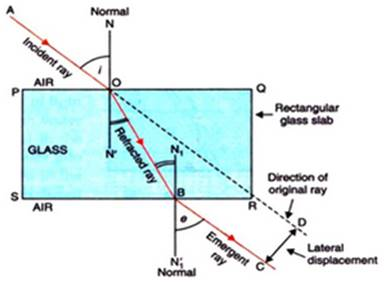### Prove the following statements. If the angle of incidence and angle of emergence of a light ray falling on a glass slab are i and e respectively, prove that, i = e.

Question 2.

Prove the following statements.

If the angle of incidence and angle of emergence of a light ray falling on a glass slab are i and e respectively, prove that, i = e.

Answer:

i. Take a rectangular glass slab PQRS as shown in below figure.

ii. Draw an incident ray AO and a normal NN’. Measure the angle of incidence i.

iii. As light ray travels from air to glass i.e. rarer to denser medium it bends towards the normal. So refracted ray OB bend towards normal.

iv. Again light ray passes from glass to air at surface SR so emergent ray BC bends away from normal N1N1’. Measure the angle e.

v. We will find that angle of incidence i = angle of emergence e. We will also observed that incident ray and emergent ray are parallel to each other since angle of incidence i = angle of emergence e.